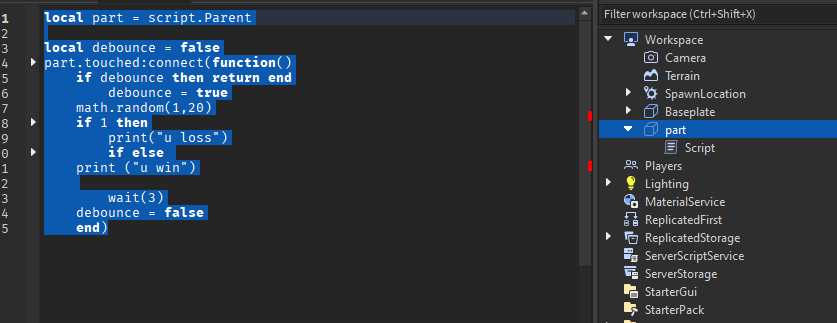# Cant script, help required

You can write your topic however you want, but you need to answer these questions:

1. What do you want to achieve? Keep it simple and clear!
I want my code to print out u loss or u win after stepping on a part but i dont want the part to be clicked 100 times in a second so i tried to use debounce but it didnt work .
2. What is the issue? Include enough details if possible!
Cant debounce script
3. What solutions have you thought of so far?
I tried editing the debounce script a little nothing chaned i think it has to do with the print script im trying to use
After that, you should include more details if you have any. Try to make your topic as descriptive as possible, so that it’s easier for people to help you!

Script: local part = script.Parent

local debounce = false
part.touched:connect(function()
if debounce then return end
debounce = true
math.random(1,20)
if 1 then
print(“u loss”)
if else
print (“u win”)

``````	wait(3)
debounce = false
end)
``````I just like shoving 232 if statements.

``````local part = script.Parent
--part

local debounce = true
part.Touched:Connect(function() --if touched then connect
if debounce == true then-- makes it happens once
debounce = false
--set it so it doesn't repeat
local randommath =  math.random(1,20)
--make a varible and get random #
if randommath == 1 then
-- if 1
print("u lost")
elseif randommath ~= 1 then
-- if not 1 so anything else
print("u win")
end
wait(3)
debounce = true
--set it back to repeat
end
end)
``````

ima shove everything since I am to lazy to explain and watch no one reply.

1 Like

I would recommend to change the debouce to debouce = {} and change the code like this:

``````local part = script.Parent
--part

local debounce = {}
part.Touched:Connect(function(hit) --if touched then connect
if debounce[hit.Parent] == nil then-- makes it happens once
debounce[hit.Parent] = part.Parent
--set it so it doesn't repeat
local randommath =  math.random(1,20)
--make a varible and get random #
if randommath == 1 then
-- if 1
print("u lost")
elseif randommath ~= 1 then
-- if not 1 so anything else
print("u win")
end